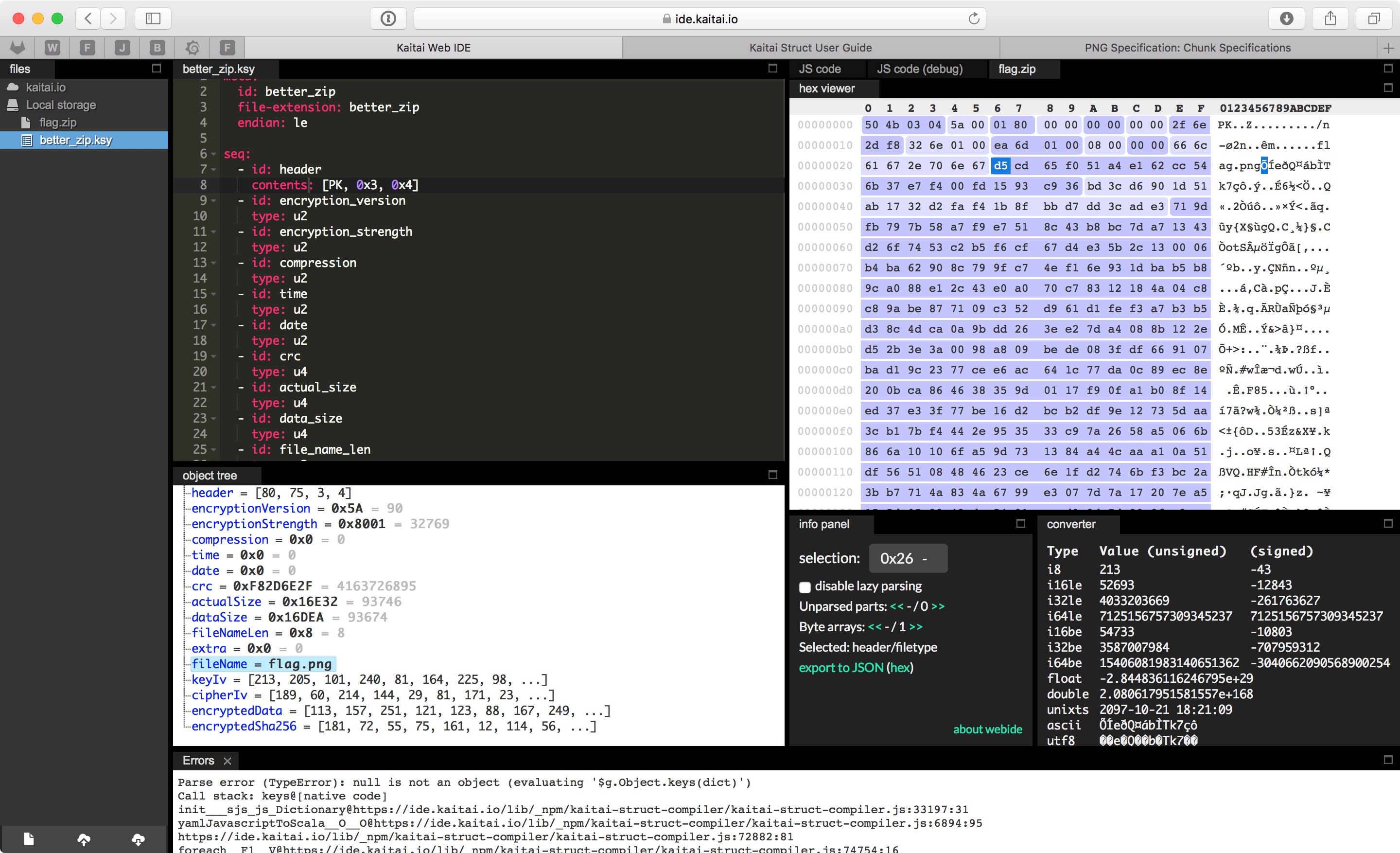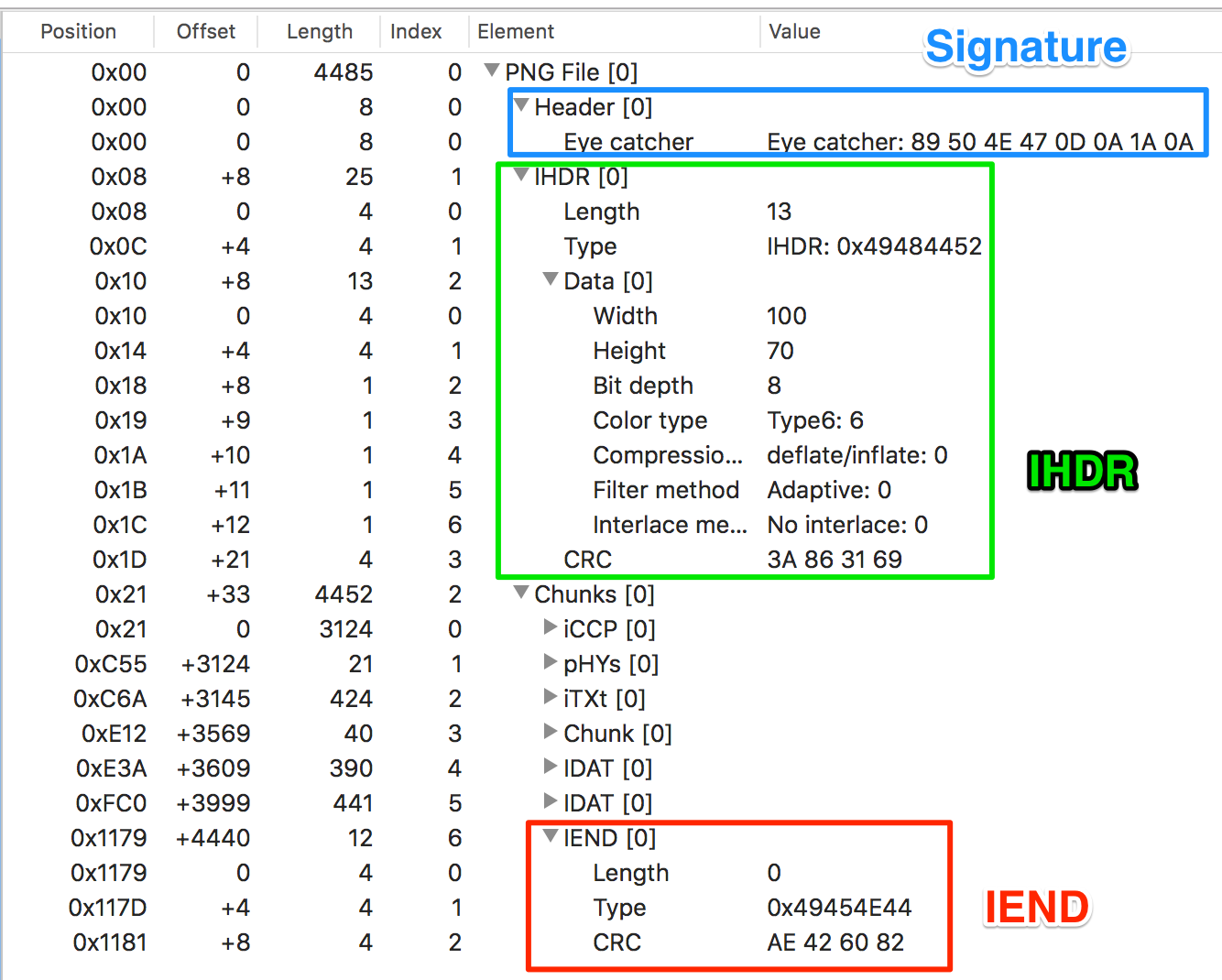# Google CTF 2018 Better Zip writeup

BETTER ZIP

The legacy ZIP crypto is long broken, so we've fixed it

Attachment

After unpacking the achive we find two files: `better_zip.py` and `flag.zip`.

`better_zip.py` — an implementation of the "better-zip" encryption for ZIP,

`flag.zip` — a ZIP file with the "better-zip" encryption.

Let's watch what's inside the `flag.zip`.

This is how this file was created according to `better_zip.py`:

``````# A super short ZIP implementation.
def SETBIT(n):
return 1 << n

def db(v):
return pack("<B", v)

def dw(v):
return pack("<H", v)

def dd(v):
return pack("<I", v)

...

# File creation
"PK\3\4",
dw(90),  # The encryption is so good it's version 9.0 at least!
dw(SETBIT(0) | SETBIT(15)),  # Super strong encryption enabled!!!
dw(0),  # No compression.
dw(0), dw(0),  # Time/date, we don't care.
dd(crc),
dd(actual_sz),
dd(len(data)),
dw(len(fname)),
dw(0),  # Extra field length.
fname
]

arc.write(encrypted_data)
arc.write(encrypted_hash)
``````

For exploring the`flag.zip` content we will use Kaitai Struct. This tool has good docs so it will be easy.

Here is what I've got for our "better-zip" format:

``````meta:
id: bzip
file-extension: bzip
endian: le

seq:
contents: [PK, 0x3, 0x4]
- id: encryption_version
type: u2
- id: encryption_strength
type: u2
- id: compression
type: u2
- id: time
type: u2
- id: date
type: u2
- id: crc
type: u4
- id: actual_size
type: u4
- id: data_size
type: u4
- id: file_name_len
type: u2
- id: extra
type: u2
- id: file_name
type: str
encoding: UTF-8
size: file_name_len

- id: key_iv
size: 20
- id: cipher_iv
size: 20
- id: encrypted_data
size: data_size
- id: encrypted_sha256
size: 32
``````

And this is how it looks in Kaitai Web IDE:As we can see there is a file with the name `flag.png` in our "better-zip" achive.

Also using `kaitai-struct-compiler` we can easily generate python parser for "better-zip" which will be useful for us later:

``````mkdir parser
cd parser
touch __init__.py
cp ../better_zip.ksy .
kaitai-struct-compiler -t python better_zip.ksy
``````

Let's move on and look closer at the encryption itself. Here is the encryption code:

``````POLY_SZ = 20

class LFSR:
def __init__(self, poly, iv, sz):
self.sz = sz
self.poly = poly
self.r = iv
self.mask = (1 << sz) - 1

def get_bit(self):
bit = (self.r >> (self.sz - 1)) & 1

new_bit = 1
for i in xrange(self.sz):
new_bit ^= (masked >> i) & 1

self.r = ((self.r << 1) | new_bit) & self.mask
return bit

class LFSRCipher:
def __init__(self, key, poly_sz=8, key_iv=None, cipher_iv=None):
if len(key) < poly_sz:
raise Exception('LFSRCipher key length must be at least %i' % poly_sz)
key = BitStream(key)

if key_iv is None:
key_iv = os.urandom(poly_sz)
self.key_iv = key_iv
key_iv_stream = BitStream(key_iv)

if cipher_iv is None:
cipher_iv = os.urandom(poly_sz)
self.cipher_iv = cipher_iv
cipher_iv_stream = BitStream(cipher_iv)

self.lfsr = []
for i in xrange(8):
l = LFSR(key.get_bits(poly_sz) ^ key_iv_stream.get_bits(poly_sz),
cipher_iv_stream.get_bits(poly_sz), poly_sz)
self.lfsr.append(l)

def get_keystream_byte(self):
b = 0
for i, l in enumerate(self.lfsr):
b |= l.get_bit() << i
return b

return self.key_iv + self.cipher_iv

def crypt(self, s):
s = bytearray(s)
for i in xrange(len(s)):
s[i] ^= self.get_keystream_byte()
return str(s)
``````

So, we have the LFSR (linear-feedback shift register) based encryption. An output of 8 20-bit long LFSRs is combined into one byte and then this byte is XORed with one byte of a plaintext. A key is 160 bits long and is used for the generation of a feedback function for each LFSR. An initial state of each LFSR is taken from `cipher_iv` sequence which is present in our "better-zip" file `flag.zip` in `cipher_iv` field.

So, we must somehow restore the feedback functions of all 8 LFSR to decrypt `flag.zip`. LFSR's length is 20 bits and it means that we can just bruteforce the feedback function if we know enough output. First 20 bits of each LFSR output are useless for us because, as we know, it is just its initial state. We can easily see it:

``````from better_zip import LFSRCipher, POLY_SZ
from parser.better_zip import BetterZip
from binascii import hexlify

# Parse file using kaitai-generated parser
bz = BetterZip.from_file('flag.zip')

c = LFSRCipher('a' * 20, POLY_SZ,
key_iv=bz.key_iv, cipher_iv=bz.cipher_iv)

dec = c.crypt(bz.encrypted_data[:20])
print hexlify(dec)
``````

An output of the script above:

``````89504e470d0a1a0a0000000d4948445200000280
``````

We already know that there is a PNG file inside the `flag.zip` and thereby we know its structure:

• PNG Signature (*) (8)
• IHDR (*)
• Length (4)
• Type (4)
• Data
• Width (4)
• Heigth (4)
• Bit depth (1)
• Color type (1)
• Compression method (1)
• Filter method (1)
• Interlace method (1)
• CRC32 (4)
• Chunks
• any of IDAT, pHYs, iCCP etc.
• IEND (*)
• Length (4)
• Type (4)
• CRC32 (4)

(*) — means required

Here is some random PNG file opened in a hex editor:We can compare our known `89504e470d0a1a0a0000000d4948445200000280` with the structure above and make sure they match.

Let's try to find another known bytes:

• first 2 bytes of height probably will be zeros because it is unlikely that the height of the picture will be more than 65535 (2 bytes);
• bit depth is 8 in most PNGs (1 byte);
• compression method is 0 according to spec (1 byte);
• filter method is 0 according to spec (1 byte);
• interlace method is more likely 0 (1 byte);
• the whole IEND chunk (12 bytes).

In total we have 18 known bytes which will be enough to narrow the number of the feedback functions variants.

Let's implement brute in C for better performance but at first we need some data for bruting:

``````# data.py
from better_zip import LFSRCipher, POLY_SZ, BitStream
from parser.better_zip import BetterZip
from binascii import hexlify, unhexlify

def xor(a, b):
c = ''
for i in range(len(a)):
c += chr(ord(a[i]) ^ ord(b[i]))
return c

# Parse file using kaitai-generated parser
bz = BetterZip.from_file('flag.zip')

png_end = unhexlify('0000000049454e44ae426082')

stream_start = xor(png_start, bz.encrypted_data[:len(png_start)])
stream_end = xor(png_end, bz.encrypted_data[-len(png_end):])

end_offset = len(bz.encrypted_data) - len(png_end)

cipher_iv_stream = BitStream(bz.cipher_iv)

print 'Stream start:', hexlify(stream_start)
print 'Stream end:', hexlify(stream_end)
print 'Stream end offset:', end_offset

for i in range(8):
print 'IV%d:' % i, cipher_iv_stream.get_bits(POLY_SZ)
``````

Output:

``````➜ python data.py
Stream start: f8cdb53e7652bdf3e7518c4ef1f439f51343d0ef7453c341fec967d4e3f6ba8f69
Stream end: f58ebdc00c8192119e9ac651
Stream end offset: 93662
IV0: 408765
IV1: 121101
IV2: 502609
IV3: 860961
IV4: 783610
IV5: 768241
IV6: 843223
IV7: 932563
``````

And here is the code of C brute:

``````// brute.c
#include <stdio.h>
#include <stdint.h>
#include <stdbool.h>
#include <string.h>
#include <stdlib.h>

#define MAX_POLY 1048576
#define OFFSET   93662

typedef struct _lfsr_t {
uint32_t poly;
uint32_t r;
uint32_t size;
uint32_t iteration;
} lfsr_t;

void lfsr_init(lfsr_t *lfsr, uint32_t poly, uint32_t iv, uint32_t size) {
lfsr->poly = poly;
lfsr->r = iv;
lfsr->size = size;
lfsr->mask = (1u << size) - 1;
lfsr->iteration = 0;
}

uint32_t lfsr_get_bit(lfsr_t *lfsr) {
uint8_t new_bit = 1;
uint32_t bit = (lfsr->r >> (lfsr->size - 1)) & 1u;

uint32_t masked = lfsr->r & lfsr->poly;

for (uint32_t i = 0; i < lfsr->size; ++i) {
new_bit ^= (masked >> i) & 1u;
}

lfsr->r = ((lfsr->r << 1u) | new_bit) & lfsr->mask;

lfsr->iteration++;

return bit;
}

uint32_t get_bit(const uint8_t *bytes, uint32_t index) {
uint32_t i_byte = index / 8;
uint32_t i_bit = 7 - index % 8;
uint32_t bit = bytes[i_byte] >> i_bit;

return bit & 1u;
}

bool check_bits(lfsr_t *lfsr, uint8_t *bytes, uint32_t bytes_len, uint32_t index) {
for (uint32_t i = index; i < bytes_len * 8; i += 8) {
if (get_bit(bytes, i) != lfsr_get_bit(lfsr)) {
return false;
}
}
return true;
}

int main() {
lfsr_t lfsr = { 0 };

uint32_t ivs[] = {
408765,
121101,
502609,
860961,
783610,
768241,
843223,
932563
};

uint8_t known_end[] = {
0xf5, 0x8e, 0xbd, 0xc0, 0x0c, 0x81, 0x92, 0x11, // IEND
0x9e, 0x9a, 0xc6, 0x51
};

uint8_t known_start[] = {
0xf8, 0xcd, 0xb5, 0x3e, 0x76, 0x52, 0xbd, 0xf3, // PNG header
0xe7, 0x51, 0x8c, 0x4e, 0xf1, 0xf4, 0x39, 0xf5, // IHDR
0x13, 0x43, 0xd0, 0xef, 0x74, 0x53, 0xc3, 0x55,
0xfe, 0xc9, 0x67, 0xd4, 0xe3, 0x6e, 0xfd, 0xcf,
0xe4
};

for (uint32_t index = 0; index < 8; index++) {

printf("[");

uint32_t offset = 7 - index;

for (uint32_t poly = 0; poly < MAX_POLY; ++poly) {

lfsr_init(&lfsr, poly, ivs[index], 20);

// Check until height low 2 bytes
if (!check_bits(&lfsr, known_start, 22, offset)) {
continue;
}

// Skip height low 2 bytes
lfsr_get_bit(&lfsr);
lfsr_get_bit(&lfsr);

// Check bit depth
if (!check_bits(&lfsr, known_start + 8 + 16, 1, offset)) {
continue;
}

// Skip color type
lfsr_get_bit(&lfsr);

// Check next 3 (most likely zeroes)
if (!check_bits(&lfsr, known_start + 8 + 18, 3, offset)) {
continue;
}

// Skip crc32
lfsr_get_bit(&lfsr);
lfsr_get_bit(&lfsr);
lfsr_get_bit(&lfsr);
lfsr_get_bit(&lfsr);

uint32_t to_skip = OFFSET - lfsr.iteration;

for (uint32_t i = 0; i < to_skip; ++i) {
lfsr_get_bit(&lfsr);
}

// Check IEND
if (check_bits(&lfsr, known_end, sizeof(known_end), offset)) {
printf("%d, ", poly);
}
}

printf("],\n");
}

return 0;
}
``````

Now we need just to brute all possible feedback functions and check that the LFSR output matches corresponding bit of our known 18 bytes.

Here is the output:

``````➜ clang -O3 brute.c -o brute
➜ ./brute
[17177, 202520, 551250, 640894, 891178, 1014778, ],
[96592, 196791, 382325, 395058, 426957, 442369, 560159, 627205, 741274, 913372, 950625, ],
[360648, 384019, 569495, 844767, 912173, ],
[51229, 80412, 161872, 501335, 696001, 787082, ],
[34375, 219426, 234454, 375028, 437605, ],
[416203, ],
[2429, 161972, 278871, 524365, 555681, 612881, 860275, 1010447, ],
[12138, 52245, 111181, 331383, 339135, 342767, 351603, 567549, 641976, 702929, 851592, ],
``````

Each row represents possible values for the feedback functions of all 8 LFSRs. Now we can just try all of these values and try to decrypt the IHDR and check its CRC:

``````# solve.py
import itertools
from better_zip import LFSRCipher, POLY_SZ, LFSR, BitStream
from parser.better_zip import BetterZip
from struct import unpack
from zlib import crc32

bz = BetterZip.from_file('flag.zip')

variants = [
[17177, 202520, 551250, 640894, 891178, 1014778, ],
[96592, 196791, 382325, 395058, 426957, 442369, 560159, 627205, 741274, 913372, 950625, ],
[360648, 384019, 569495, 844767, 912173, ],
[51229, 80412, 161872, 501335, 696001, 787082, ],
[34375, 219426, 234454, 375028, 437605, ],
[416203, ],
[2429, 161972, 278871, 524365, 555681, 612881, 860275, 1010447, ],
[12138, 52245, 111181, 331383, 339135, 342767, 351603, 567549, 641976, 702929, 851592, ],
]

c = LFSRCipher('a' * 20, POLY_SZ,
key_iv=bz.key_iv, cipher_iv=bz.cipher_iv)

for x in itertools.product(*variants):
c.lfsr = []

cipher_iv_stream = BitStream(bz.cipher_iv)
for i in range(8):
c.lfsr.append(LFSR(x[i], cipher_iv_stream.get_bits(POLY_SZ), POLY_SZ))

dec = c.crypt(bz.encrypted_data[:33])

crc32_expected = crc32(dec[12:-4]) & 0xffffffffL
crc32_actual = unpack('>I', dec[-4:])

if crc32_actual == crc32_expected:
print 'Success!', x

rest = c.crypt(bz.encrypted_data[33:])

with open('flag.png', 'wb') as f:
f.write(dec + rest)

break
``````

Output:

``````➜ python solve.py
Success! (891178, 96592, 360648, 51229, 219426, 416203, 161972, 342767)
``````

Finally we get the flag: